Courses
Courses for Kids
Free study material
Offline Centres
More

# Construction of a SquareLast updated date: 07th Dec 2023
Total views: 303.9k
Views today: 7.03k## Introduction to Construction of a Square

In Geometry, a square is a two-dimensional figure with four equal sides and four equal angles. Each angle of the square measures 90 degrees. Also, the diagonals of a square are equal and bisect each other at 90 degrees. The square is considered a special type of rectangle as all the properties of the square are quite similar to the properties of a rectangle. The only difference between the two is that the rectangle has only its opposite sides equal.  Hence, the rectangle will be called a square if the length of all its four sides is equal.

Read the article below to learn the steps of construction of a square with a compass and a ruler and steps of construction of a square inscribed in a  circle.

## Construction of a Square with Compass and Ruler

A square is a closed, two-dimensional figure with four equal sides. The four angles of a square are also equal i.e. 90 degrees each. The only dimension we have been provided is the length of one side of a square i.e. 5 cm.  We know that the interior angles of a square are at right angles or equal to 90 degrees. Hence, we do not require any other measurement for constructing a square. The measurement of the length of all the four sides of a square equal and perpendicular to each other. With the help of this information, we can now construct a square each of whose sides measure 5 cm.

### Construction of a Square with a Given Side of 5 cm

Below are the steps for constructing a square with a compass whose each side measure 5 cm:

1. Draw a line segment AB of 5 cm with the help of the ruler.1. Extend the line segment AB. Place the pointer of the compass on point B, and with convenient width, draw two arcs on each side of B as shown in the figure given below. Mark the point as F and G.1. With F as the center and the radius 5 cm draw an arc above point B.

2. With G as a center and the same radius of the compass, draw on the arc above point B, crossing the previous arc. Mark the point H, where both the arcs meet as shown in the figure below.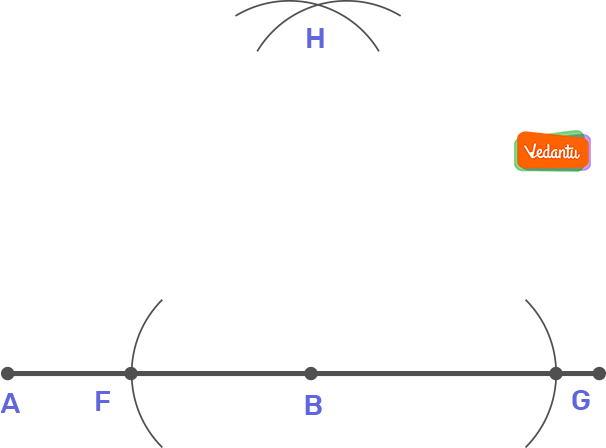1. Join point B and H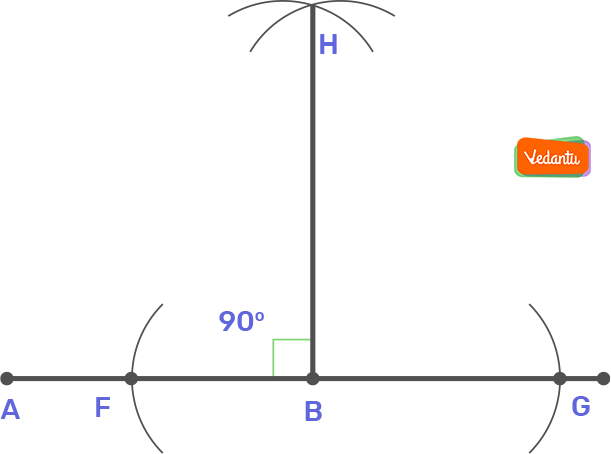1. Taking A as the center and radius 5 cm, draw an arc above the point A.

2. Now with the same radius and taking B as center, draw an arc across BH as shown in the figure below, and mark the point as C. This is the vertex of a square.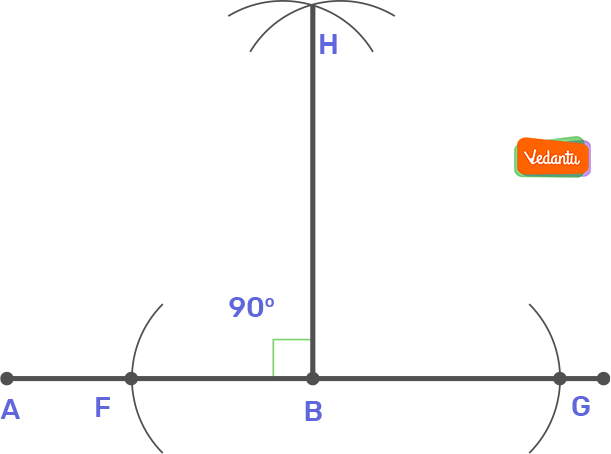1. Now with the same radius and taking C as the center, draw an arc to the left of C, crossing the previous arc. Mark the point D where both the arc meets.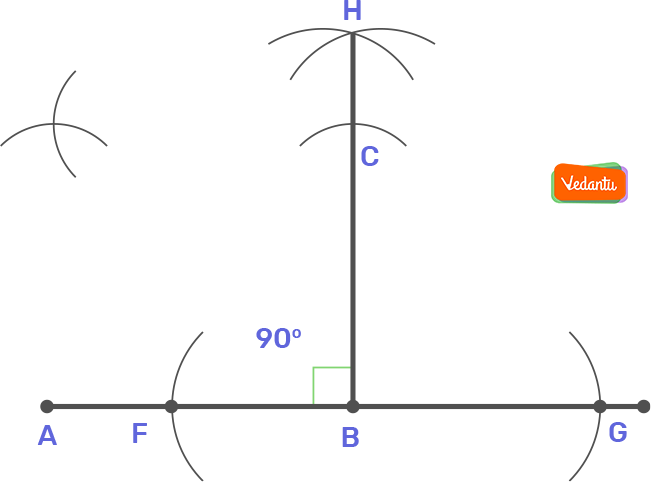1. Join the point CD and AD to get the desired square ABCD of each side 5 cm.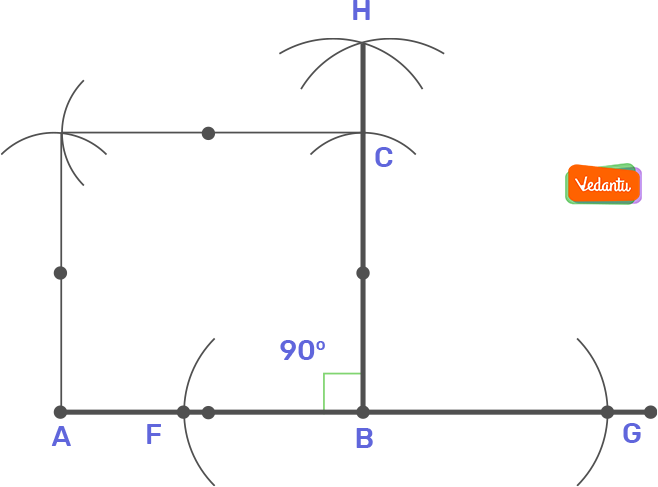## Square Inscribed in a Circle

A square is drawn inside a circle or inscribed in a circle if its four vertices lie on the circumference of a circle. The diagonals of a square are always equal to the diameter of a circle. The figure given below shows the square inscribed in a circle.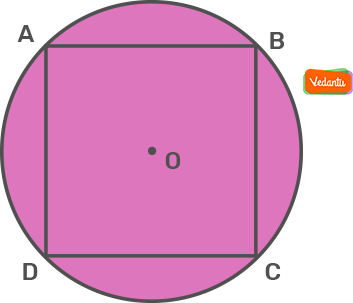### Construction of a Square Inscribed in a Circle

Following  are the steps to construct a square inscribed in a circle

1. Draw a circle with center O with the help of the compass.

2. Mark a point A on the edge of the circle as shown in the figure given below. This will be considered as one of the vertices of a square.

3. Using the ruler, draw the diameter of a circle from point A that passes through the center of the circle, creating a new ending point C.

4. Taking A as center and radius more than half of the length AC, draw an arc both above and below the point O.

5. Taking C as center and with the same radius, draw an arc both above and below the point O that will intersect the arc drawn in step 5.

6. Draw a line through the point where opposite arcs meet each other. Extends the line long enough so that it touches the circle both at the top and bottom creating the new points B and D. The line segment BD will also be the diameter of the circle.

7. Join each successive point i.e. A, B, C, and D to form a square as shown in the figure below.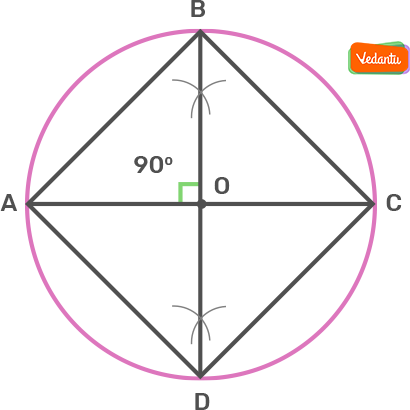Hence, you will get a square inscribed in the given circle.

### Solved Examples

1. A square with side length a is inscribed in a circle. Determine the radius of a circle in terms of the side length of square a.

Solution:

The diagonals of a square are the diameter of a circle. Let d and r be the diameter and radius of a circle respectively. According to the Pythagorean theorem, we have

d² = a² + a²

d² = 2a²

D = $\sqrt{2a^{2}}$

= $a\sqrt{2}$

As we know, the diameter of a circle is twice its radius. So

R = d/2

= $a\sqrt{2}/2$

2. Construct a square ABCD whose measurement of the length of the diagonals is 6 cm.  Measure the sides of a  square.

Solution:

As we know, all four sides of a square are equal, and also each angle of the square measures 90 degrees each. We also know that the diagonals of a square are equal and perpendicularly bisect to each other.

With these properties, we can construct a square whose diagonal is 6 cm in length. Following are the steps to construct a square whose diagonal is 6 cm.

1. Draw a line segment AC of 6 cm with the help of the ruler.

2. Construct a perpendicular bisector XY through the midpoint of AB.

3. The perpendicular bisector XY will intersect the line segment AC at O. We get OC = OA= 3 cm.

4. Taking O as center and radius 3.cm, draw two arcs cutting the line XY at points B and D.

5. Join the line segments AB, BC, CD, and DA. Hence, ABCD is the desired square of diagonal 6 cm as shown below.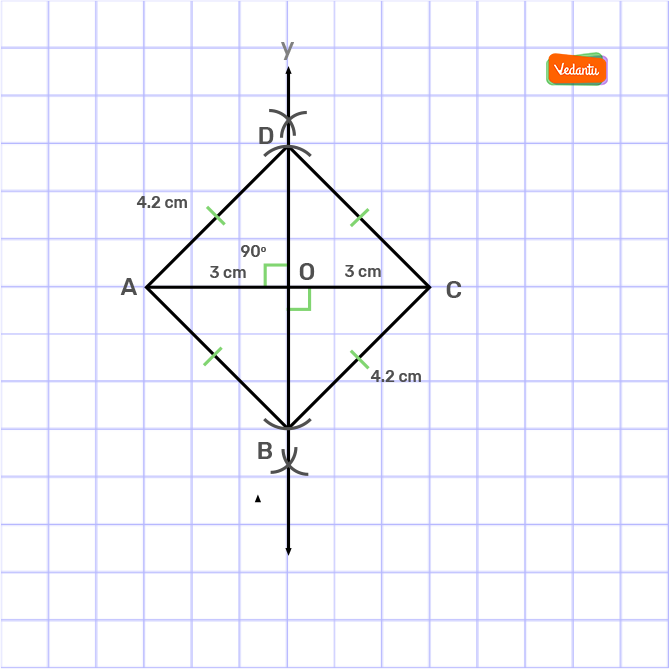Measuring  the sides of a square using a ruler,  we get AB = BC = CD = DA = 4.2 cm.

## FAQs on Construction of a Square

1. What are the Important Properties of a Square?

Ans: Some important properties of a square are discussed below:

• The length of all four sides of a square is equal.

• The length of the sides of a square opposite to each other are equal and parallel.

• The measurement of all four interior angles is equal to 90 degrees.

• The length of the diagonals of a square is also more than the length of its sides.

• The two diagonals of a square are equal to each other and bisect each other at 90 degrees.

• Each diagonal of a square divides the square into two similar isosceles triangles.

2. What are the Properties of a Square Inscribed in a Circle?

Ans: Following are the properties of a square inscribed in a circle

• The two diagonals of an inscribed square meet at the center point of the circumcircle.

• The diagonals of an inscribed square are always equal to the diameter of a circle

• The circumscribed circle of squares is formed with the four vertices of a square.

• The radius of a square is equal to the radius of the circumcircle of a square.

• The diagonals of an inscribed square are 2 times the length of the sides of a square.